Скачать презентацию Data Security and Encryption CSE 348 1

cacb4170a1a0ec0b8e29651d04c763fc.ppt

• Количество слайдов: 68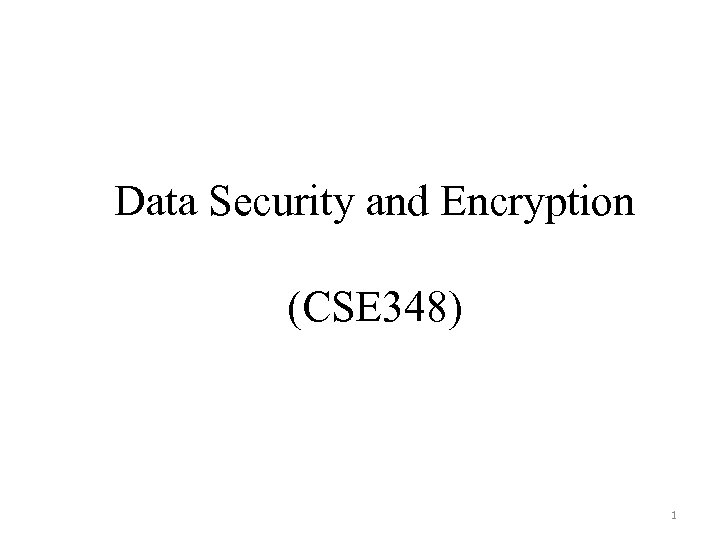Data Security and Encryption (CSE 348) 1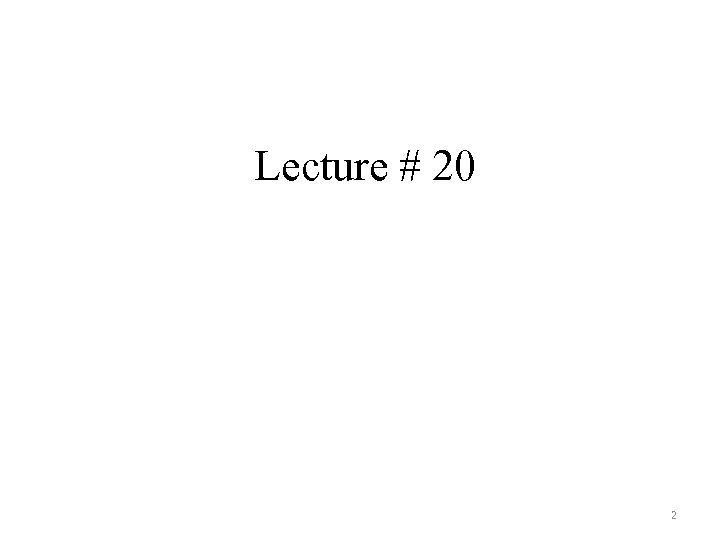Lecture # 20 2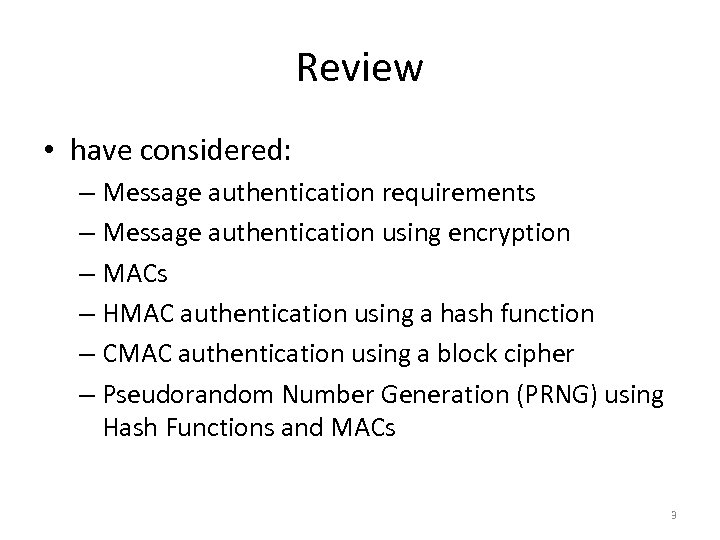Review • have considered: – Message authentication requirements – Message authentication using encryption – MACs – HMAC authentication using a hash function – CMAC authentication using a block cipher – Pseudorandom Number Generation (PRNG) using Hash Functions and MACs 3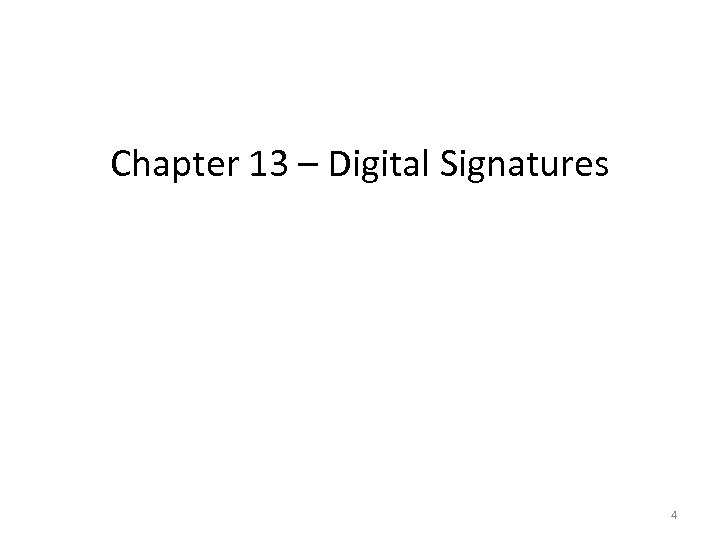Chapter 13 – Digital Signatures 4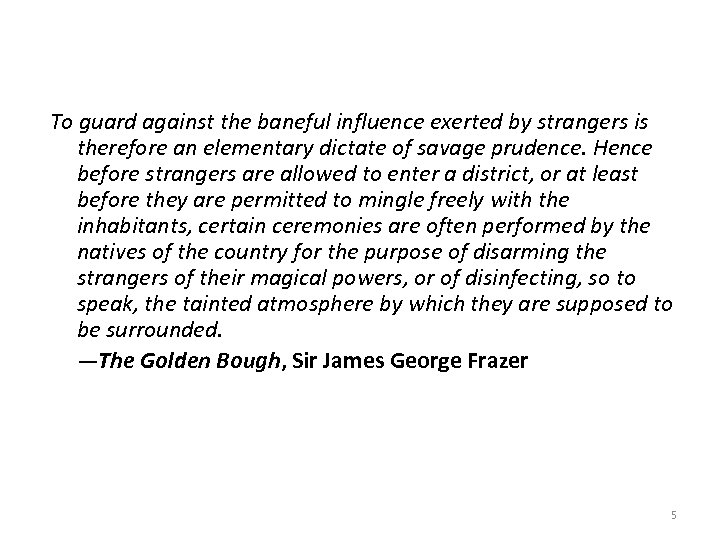To guard against the baneful influence exerted by strangers is therefore an elementary dictate of savage prudence. Hence before strangers are allowed to enter a district, or at least before they are permitted to mingle freely with the inhabitants, certain ceremonies are often performed by the natives of the country for the purpose of disarming the strangers of their magical powers, or of disinfecting, so to speak, the tainted atmosphere by which they are supposed to be surrounded. —The Golden Bough, Sir James George Frazer 5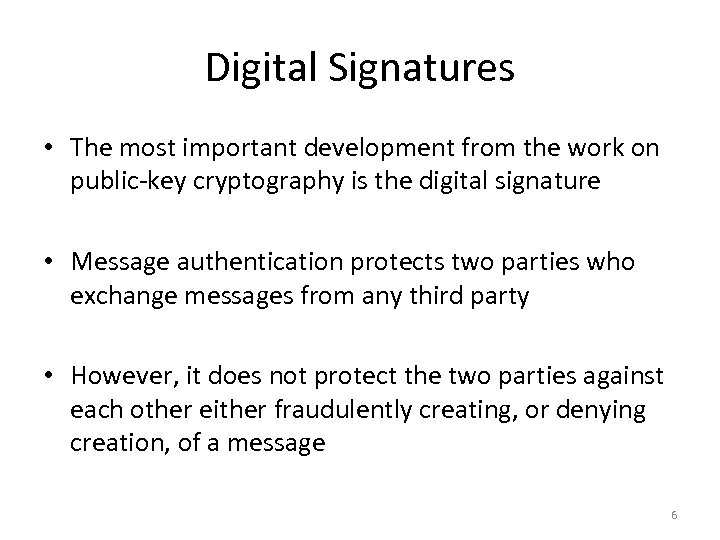Digital Signatures • The most important development from the work on public-key cryptography is the digital signature • Message authentication protects two parties who exchange messages from any third party • However, it does not protect the two parties against each other either fraudulently creating, or denying creation, of a message 6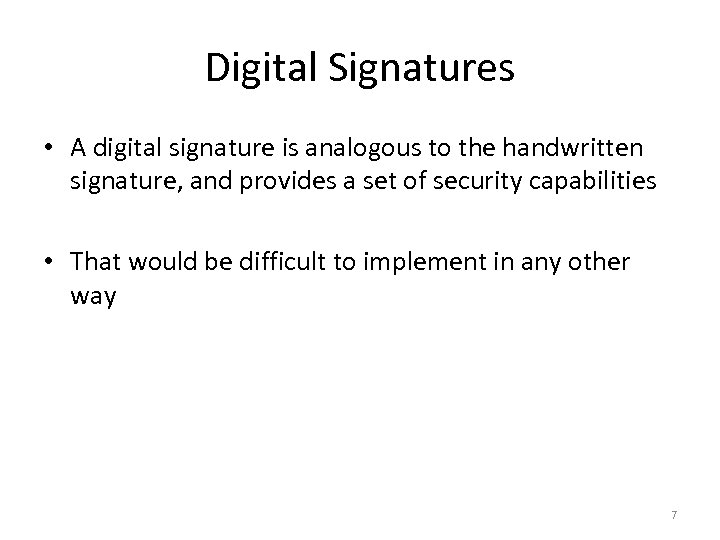Digital Signatures • A digital signature is analogous to the handwritten signature, and provides a set of security capabilities • That would be difficult to implement in any other way 7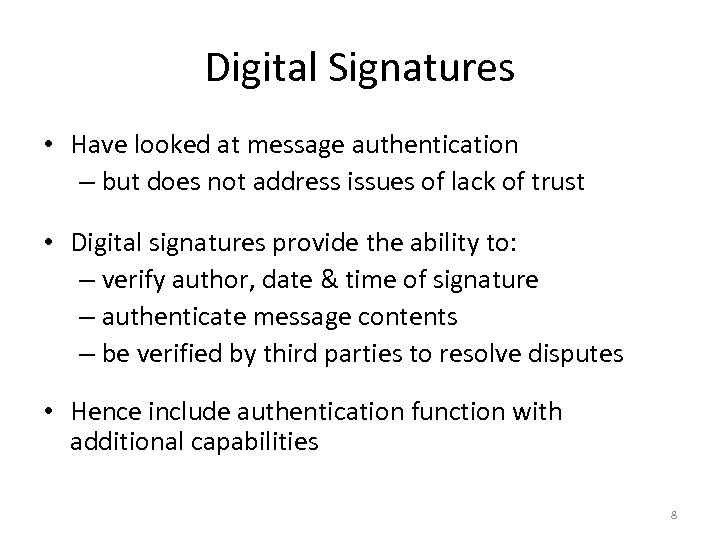Digital Signatures • Have looked at message authentication – but does not address issues of lack of trust • Digital signatures provide the ability to: – verify author, date & time of signature – authenticate message contents – be verified by third parties to resolve disputes • Hence include authentication function with additional capabilities 8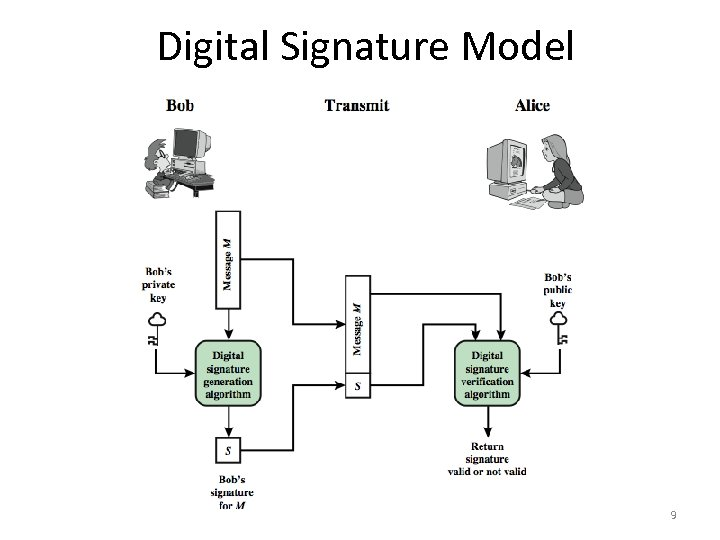Digital Signature Model 9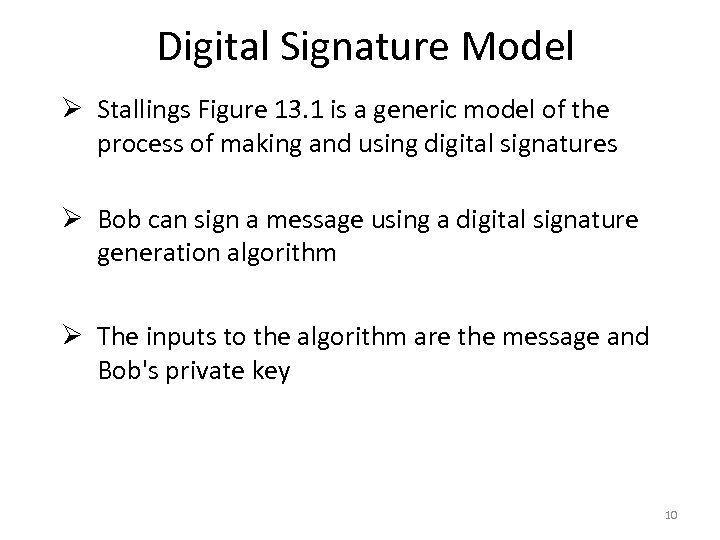Digital Signature Model Ø Stallings Figure 13. 1 is a generic model of the process of making and using digital signatures Ø Bob can sign a message using a digital signature generation algorithm Ø The inputs to the algorithm are the message and Bob's private key 10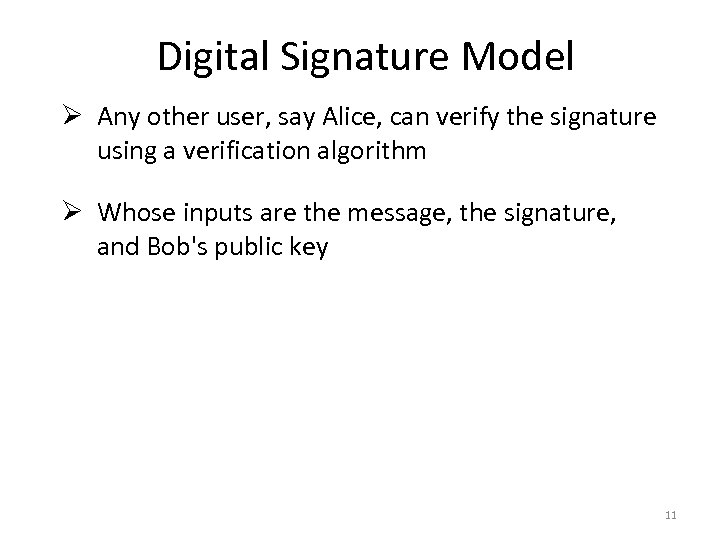Digital Signature Model Ø Any other user, say Alice, can verify the signature using a verification algorithm Ø Whose inputs are the message, the signature, and Bob's public key 11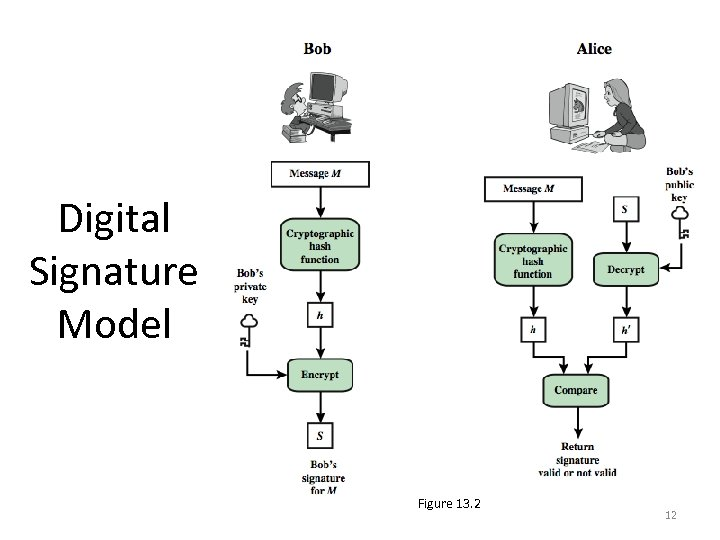Digital Signature Model Figure 13. 2 12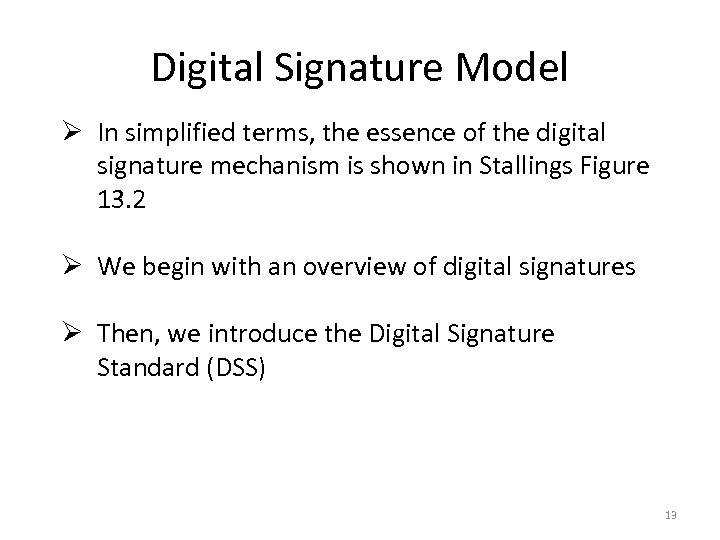Digital Signature Model Ø In simplified terms, the essence of the digital signature mechanism is shown in Stallings Figure 13. 2 Ø We begin with an overview of digital signatures Ø Then, we introduce the Digital Signature Standard (DSS) 13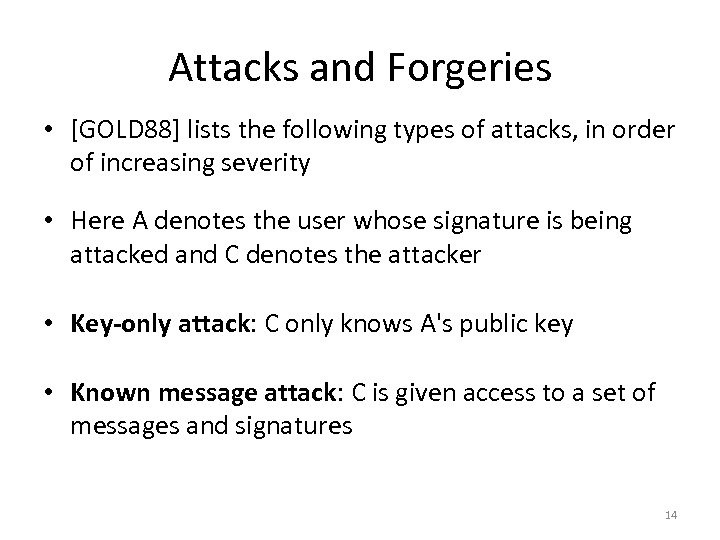Attacks and Forgeries • [GOLD 88] lists the following types of attacks, in order of increasing severity • Here A denotes the user whose signature is being attacked and C denotes the attacker • Key-only attack: C only knows A's public key • Known message attack: C is given access to a set of messages and signatures 14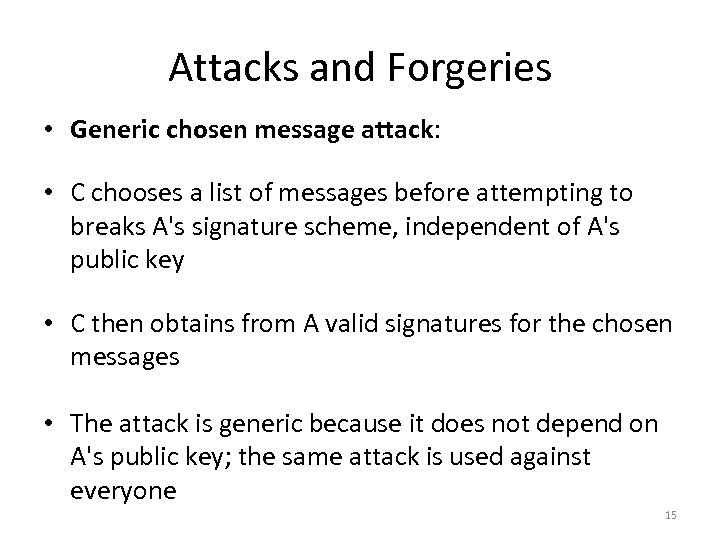Attacks and Forgeries • Generic chosen message attack: • C chooses a list of messages before attempting to breaks A's signature scheme, independent of A's public key • C then obtains from A valid signatures for the chosen messages • The attack is generic because it does not depend on A's public key; the same attack is used against everyone 15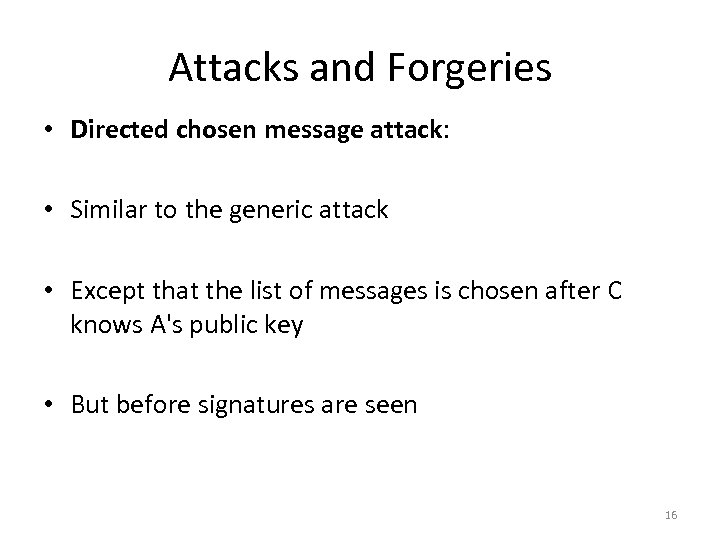Attacks and Forgeries • Directed chosen message attack: • Similar to the generic attack • Except that the list of messages is chosen after C knows A's public key • But before signatures are seen 16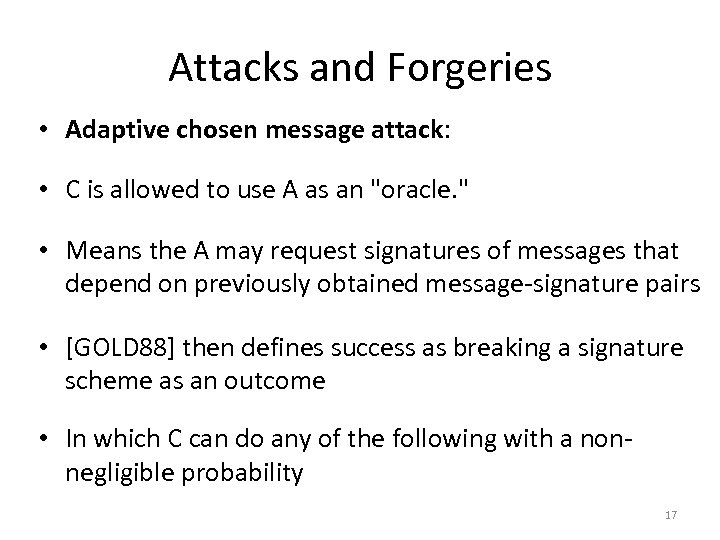Attacks and Forgeries • Adaptive chosen message attack: • C is allowed to use A as an "oracle. " • Means the A may request signatures of messages that depend on previously obtained message-signature pairs • [GOLD 88] then defines success as breaking a signature scheme as an outcome • In which C can do any of the following with a nonnegligible probability 17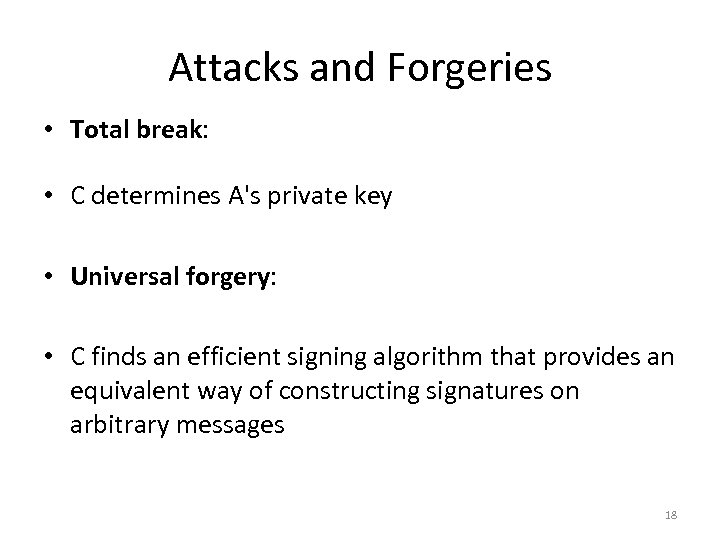Attacks and Forgeries • Total break: • C determines A's private key • Universal forgery: • C finds an efficient signing algorithm that provides an equivalent way of constructing signatures on arbitrary messages 18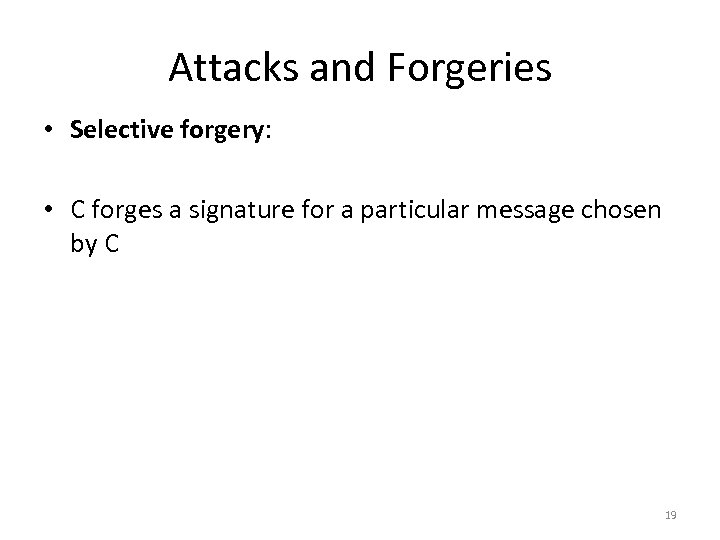Attacks and Forgeries • Selective forgery: • C forges a signature for a particular message chosen by C 19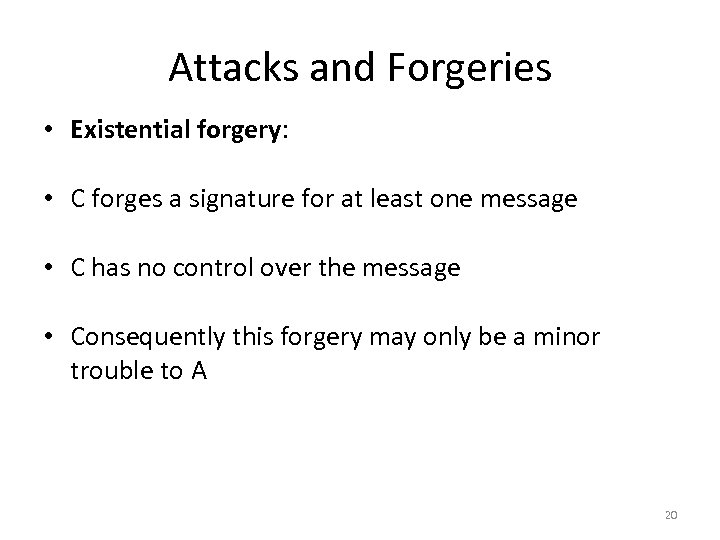Attacks and Forgeries • Existential forgery: • C forges a signature for at least one message • C has no control over the message • Consequently this forgery may only be a minor trouble to A 20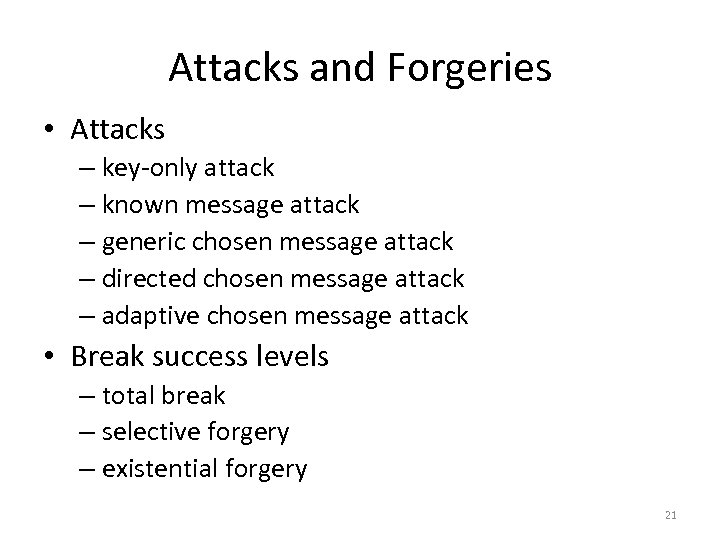Attacks and Forgeries • Attacks – key-only attack – known message attack – generic chosen message attack – directed chosen message attack – adaptive chosen message attack • Break success levels – total break – selective forgery – existential forgery 21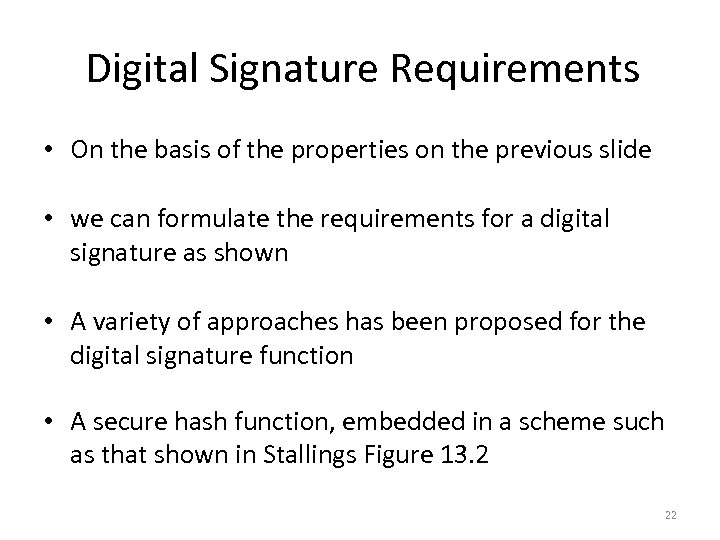Digital Signature Requirements • On the basis of the properties on the previous slide • we can formulate the requirements for a digital signature as shown • A variety of approaches has been proposed for the digital signature function • A secure hash function, embedded in a scheme such as that shown in Stallings Figure 13. 2 22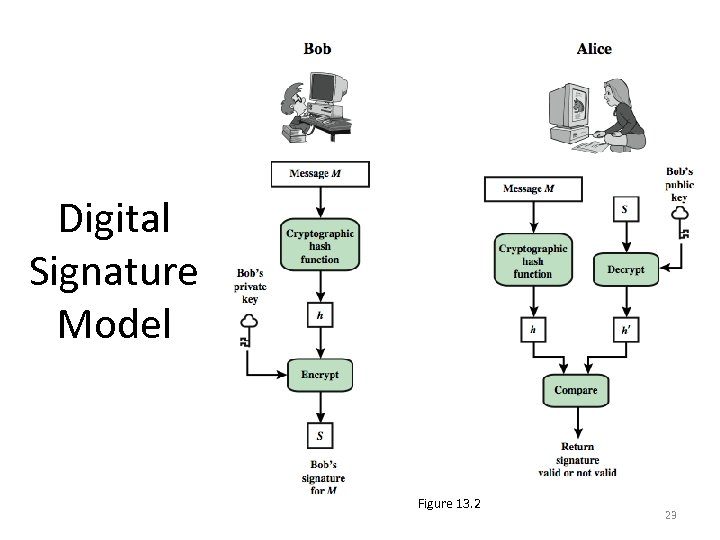Digital Signature Model Figure 13. 2 23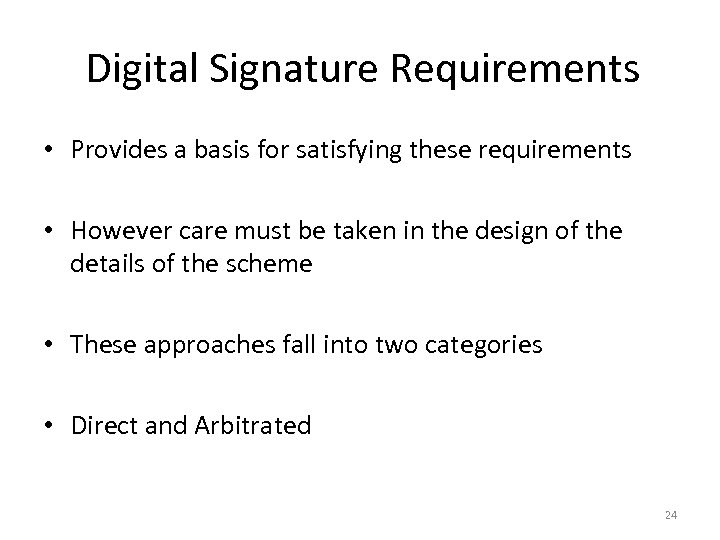Digital Signature Requirements • Provides a basis for satisfying these requirements • However care must be taken in the design of the details of the scheme • These approaches fall into two categories • Direct and Arbitrated 24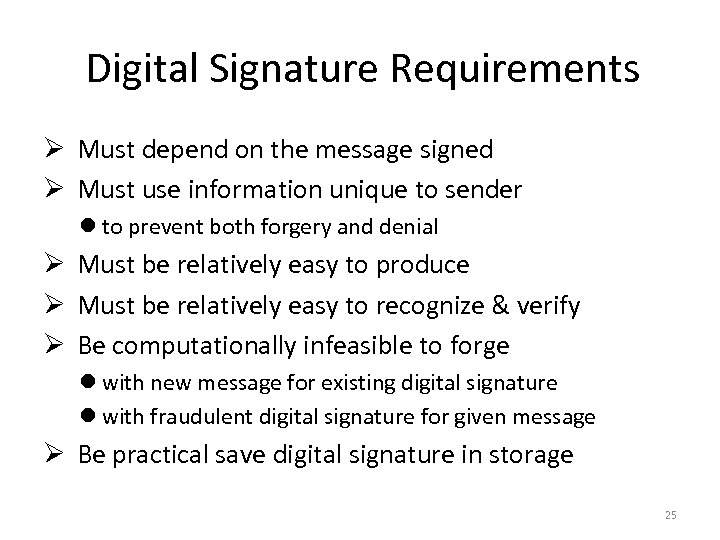Digital Signature Requirements Ø Must depend on the message signed Ø Must use information unique to sender l to prevent both forgery and denial Ø Must be relatively easy to produce Ø Must be relatively easy to recognize & verify Ø Be computationally infeasible to forge l with new message for existing digital signature l with fraudulent digital signature for given message Ø Be practical save digital signature in storage 25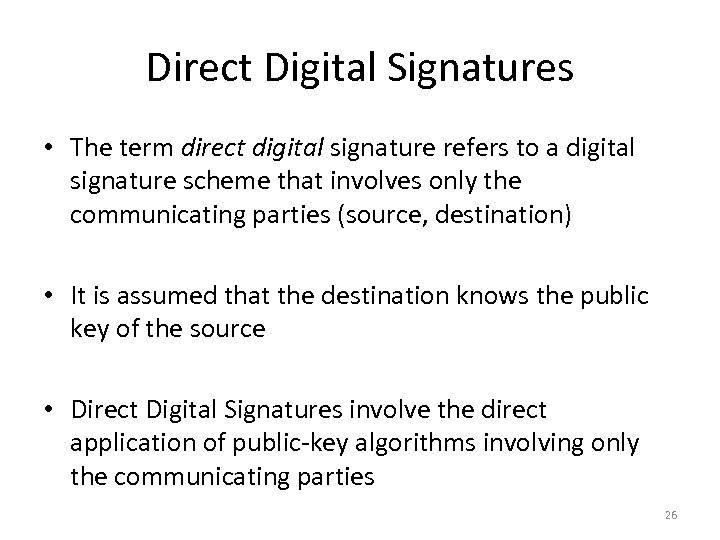Direct Digital Signatures • The term direct digital signature refers to a digital signature scheme that involves only the communicating parties (source, destination) • It is assumed that the destination knows the public key of the source • Direct Digital Signatures involve the direct application of public-key algorithms involving only the communicating parties 26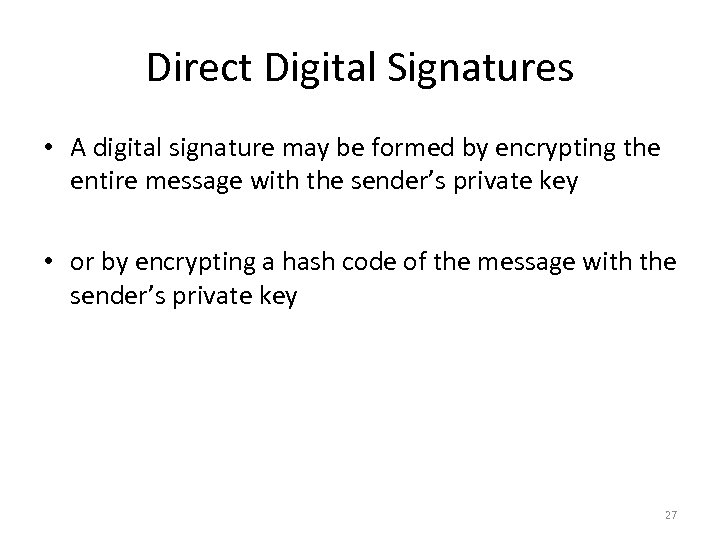Direct Digital Signatures • A digital signature may be formed by encrypting the entire message with the sender’s private key • or by encrypting a hash code of the message with the sender’s private key 27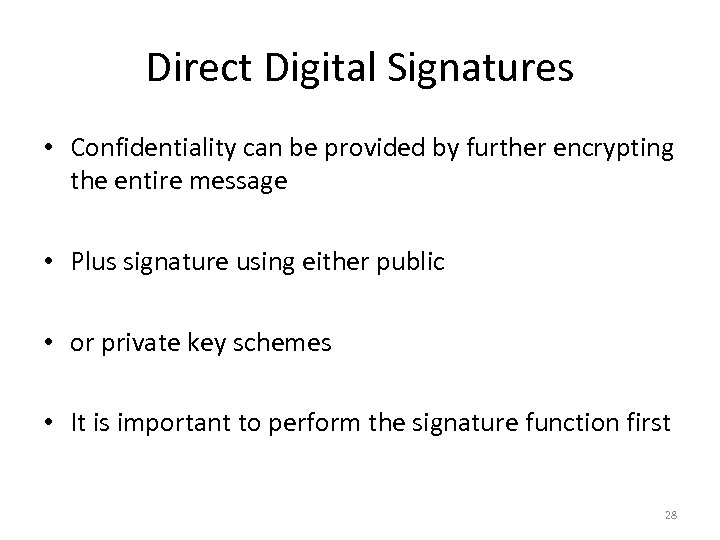Direct Digital Signatures • Confidentiality can be provided by further encrypting the entire message • Plus signature using either public • or private key schemes • It is important to perform the signature function first 28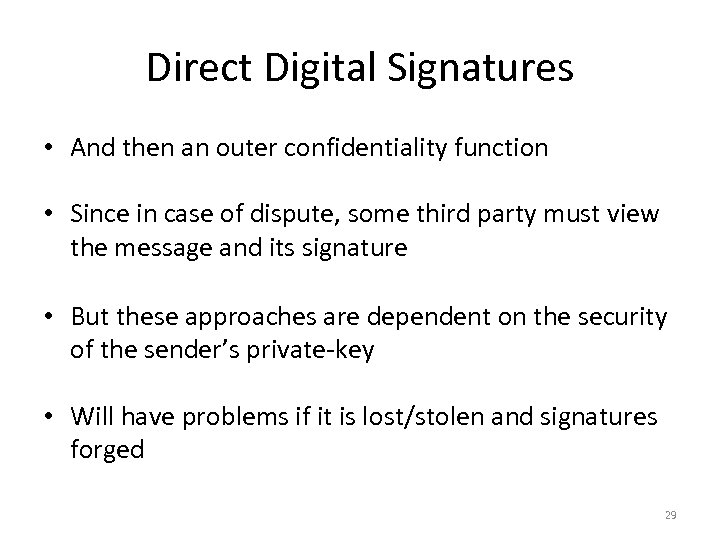Direct Digital Signatures • And then an outer confidentiality function • Since in case of dispute, some third party must view the message and its signature • But these approaches are dependent on the security of the sender’s private-key • Will have problems if it is lost/stolen and signatures forged 29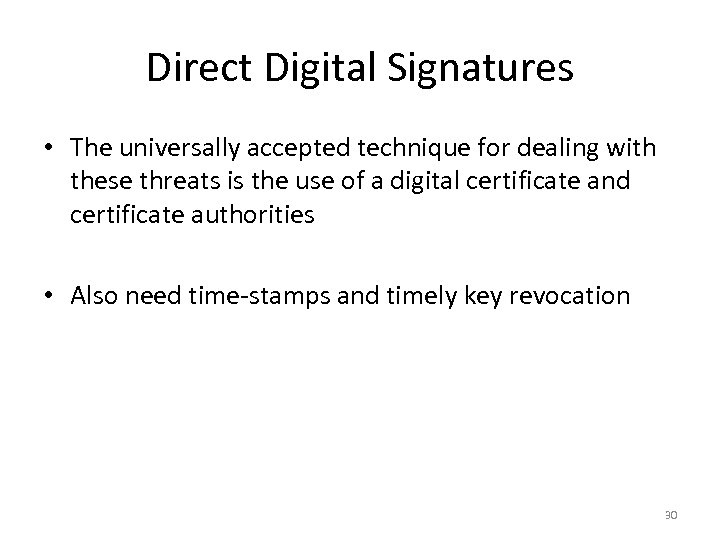Direct Digital Signatures • The universally accepted technique for dealing with these threats is the use of a digital certificate and certificate authorities • Also need time-stamps and timely key revocation 30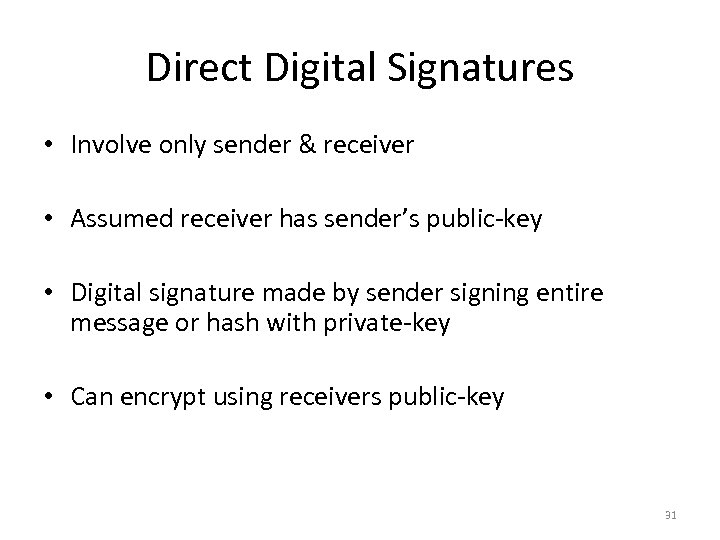Direct Digital Signatures • Involve only sender & receiver • Assumed receiver has sender’s public-key • Digital signature made by sender signing entire message or hash with private-key • Can encrypt using receivers public-key 31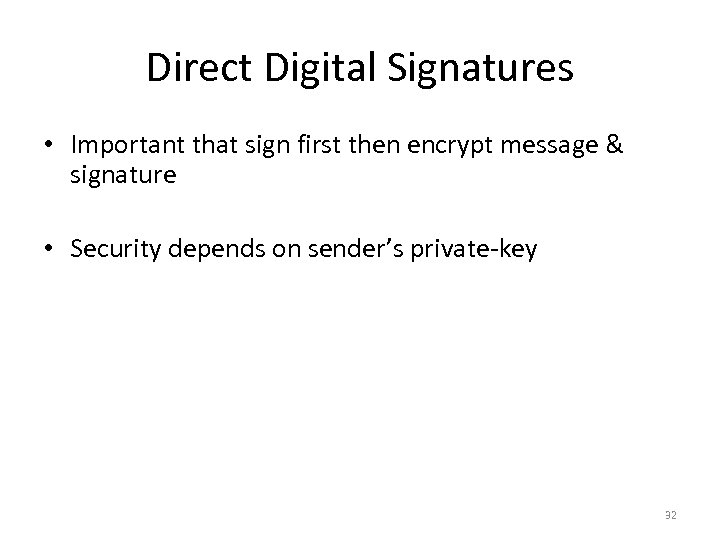Direct Digital Signatures • Important that sign first then encrypt message & signature • Security depends on sender’s private-key 32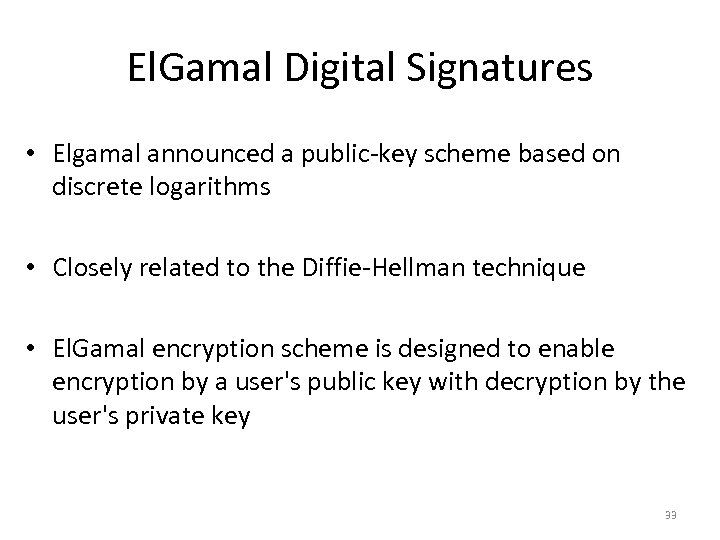El. Gamal Digital Signatures • Elgamal announced a public-key scheme based on discrete logarithms • Closely related to the Diffie-Hellman technique • El. Gamal encryption scheme is designed to enable encryption by a user's public key with decryption by the user's private key 33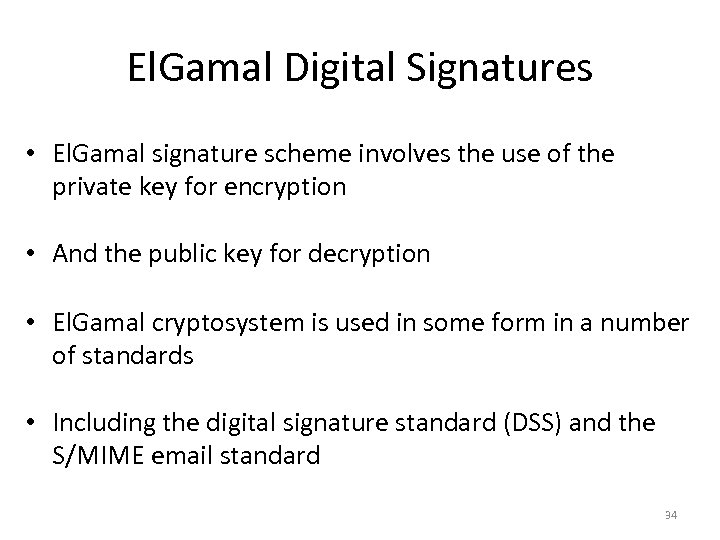El. Gamal Digital Signatures • El. Gamal signature scheme involves the use of the private key for encryption • And the public key for decryption • El. Gamal cryptosystem is used in some form in a number of standards • Including the digital signature standard (DSS) and the S/MIME email standard 34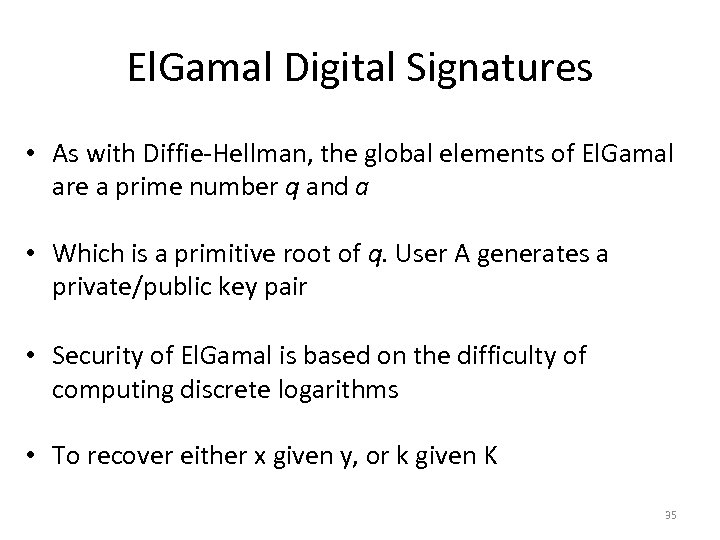El. Gamal Digital Signatures • As with Diffie-Hellman, the global elements of El. Gamal are a prime number q and a • Which is a primitive root of q. User A generates a private/public key pair • Security of El. Gamal is based on the difficulty of computing discrete logarithms • To recover either x given y, or k given K 35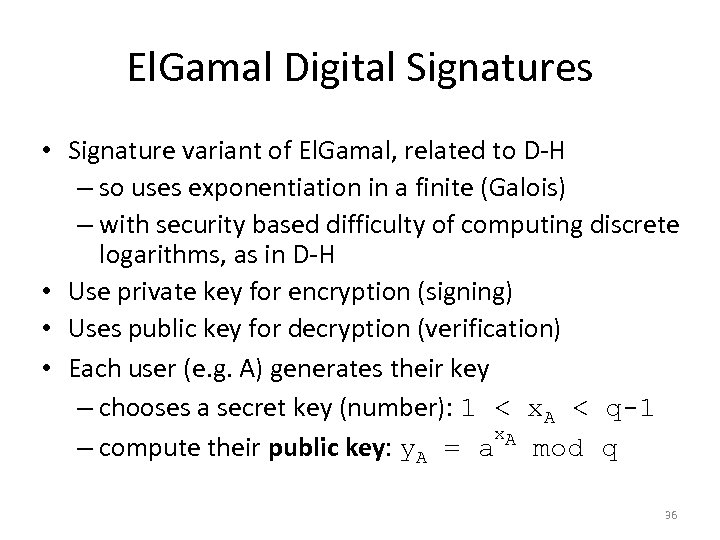El. Gamal Digital Signatures • Signature variant of El. Gamal, related to D-H – so uses exponentiation in a finite (Galois) – with security based difficulty of computing discrete logarithms, as in D-H • Use private key for encryption (signing) • Uses public key for decryption (verification) • Each user (e. g. A) generates their key – chooses a secret key (number): 1 < x. A < q-1 x. A – compute their public key: y. A = a mod q 36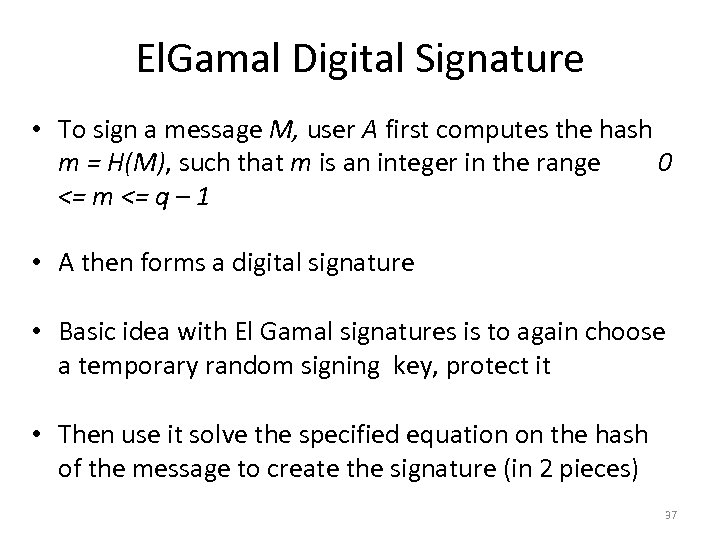El. Gamal Digital Signature • To sign a message M, user A first computes the hash m = H(M), such that m is an integer in the range 0 <= m <= q – 1 • A then forms a digital signature • Basic idea with El Gamal signatures is to again choose a temporary random signing key, protect it • Then use it solve the specified equation on the hash of the message to create the signature (in 2 pieces) 37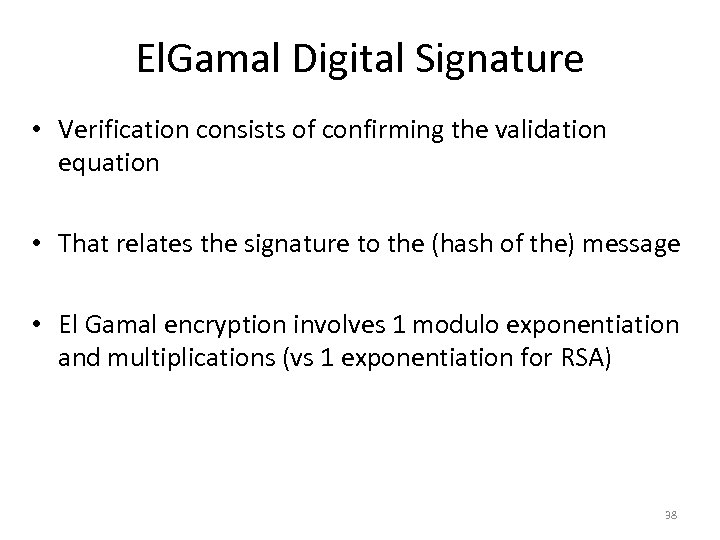El. Gamal Digital Signature • Verification consists of confirming the validation equation • That relates the signature to the (hash of the) message • El Gamal encryption involves 1 modulo exponentiation and multiplications (vs 1 exponentiation for RSA) 38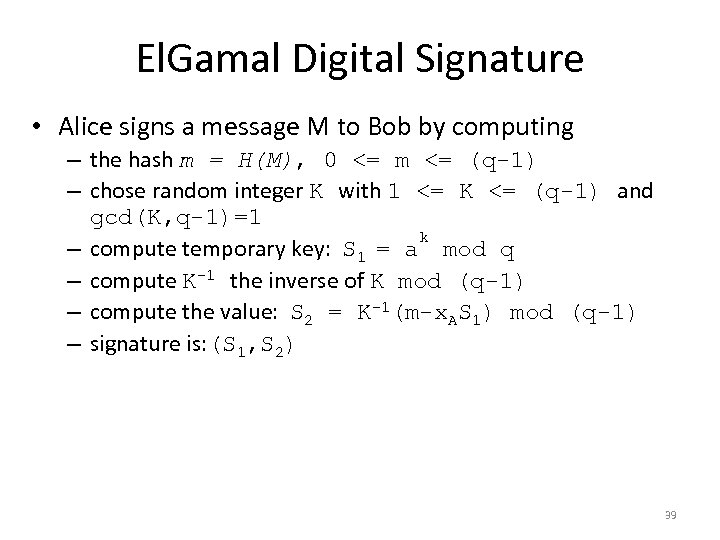El. Gamal Digital Signature • Alice signs a message M to Bob by computing – the hash m = H(M), 0 <= m <= (q-1) – chose random integer K with 1 <= K <= (q-1) and gcd(K, q-1)=1 k – compute temporary key: S 1 = a mod q – compute K-1 the inverse of K mod (q-1) – compute the value: S 2 = K-1(m-x. AS 1) mod (q-1) – signature is: (S 1, S 2) 39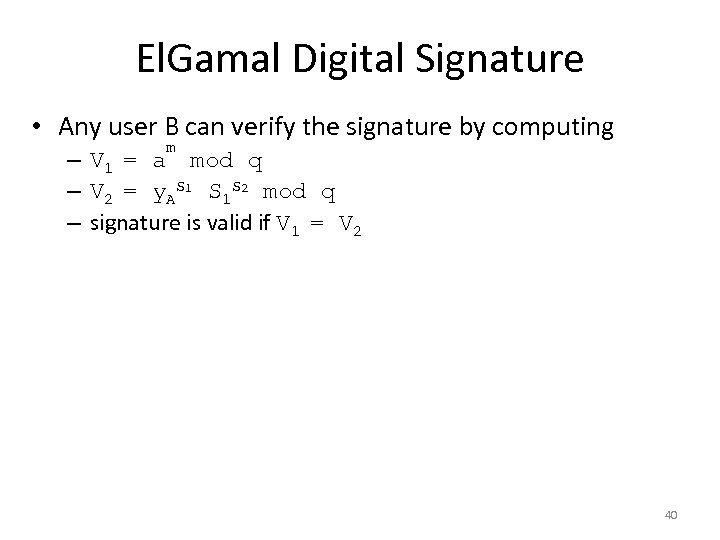El. Gamal Digital Signature • Any user B can verify the signature by computing m – V 1 = a mod q – V 2 = y. AS 1 S 1 S 2 mod q – signature is valid if V 1 = V 2 40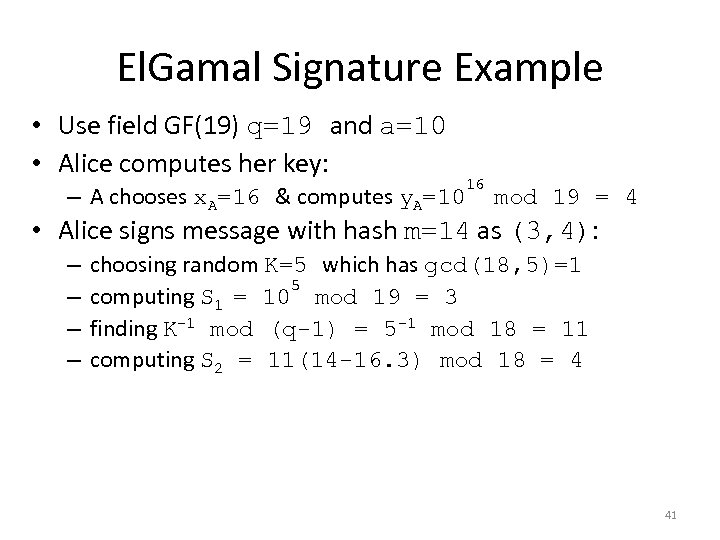El. Gamal Signature Example • Use field GF(19) q=19 and a=10 • Alice computes her key: – A chooses x. A=16 & computes y. A=10 16 mod 19 = 4 • Alice signs message with hash m=14 as (3, 4): – – choosing random K=5 which has gcd(18, 5)=1 5 computing S 1 = 10 mod 19 = 3 finding K-1 mod (q-1) = 5 -1 mod 18 = 11 computing S 2 = 11(14 -16. 3) mod 18 = 4 41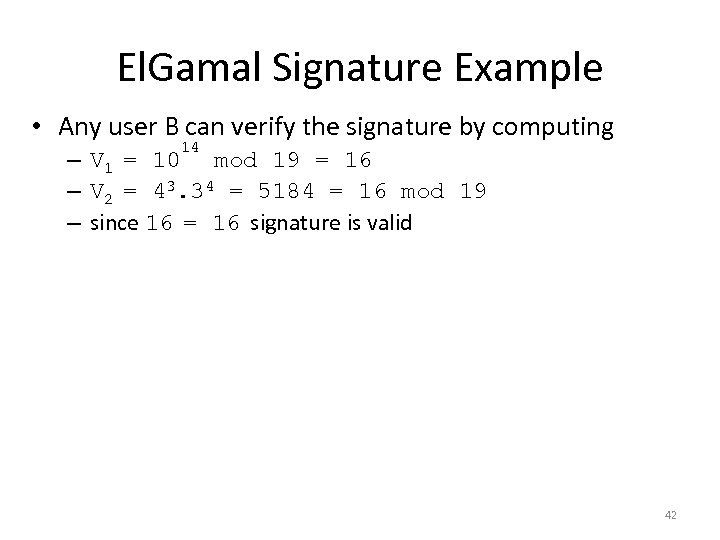El. Gamal Signature Example • Any user B can verify the signature by computing 14 – V 1 = 10 mod 19 = 16 – V 2 = 43. 34 = 5184 = 16 mod 19 – since 16 = 16 signature is valid 42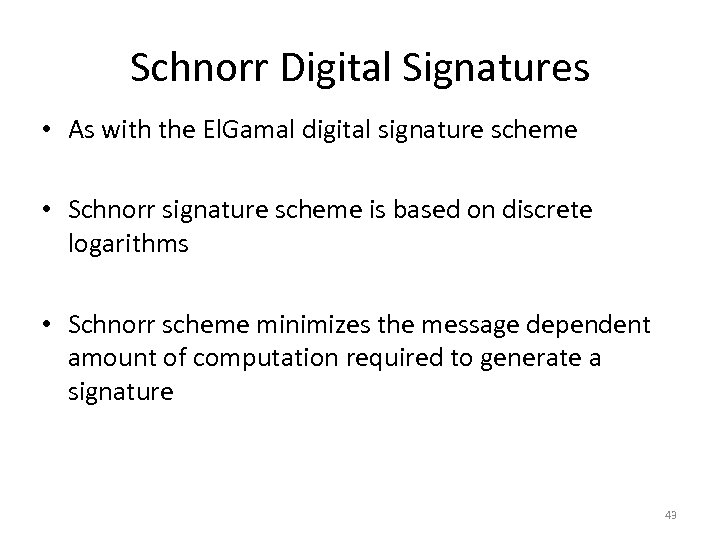Schnorr Digital Signatures • As with the El. Gamal digital signature scheme • Schnorr signature scheme is based on discrete logarithms • Schnorr scheme minimizes the message dependent amount of computation required to generate a signature 43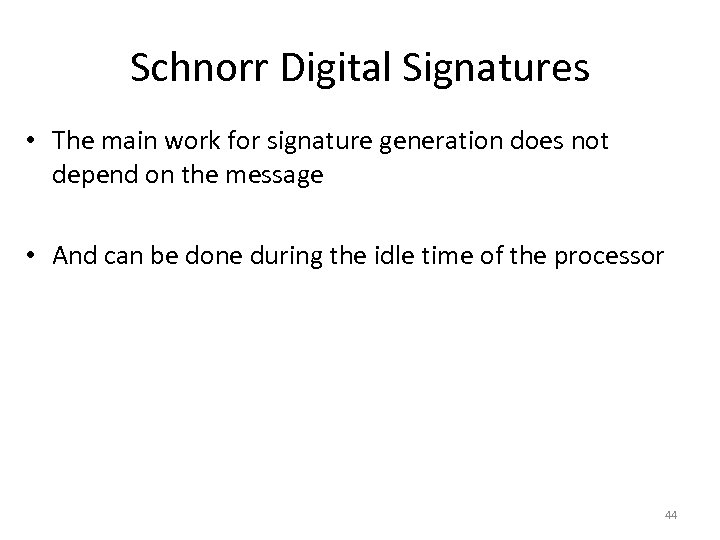Schnorr Digital Signatures • The main work for signature generation does not depend on the message • And can be done during the idle time of the processor 44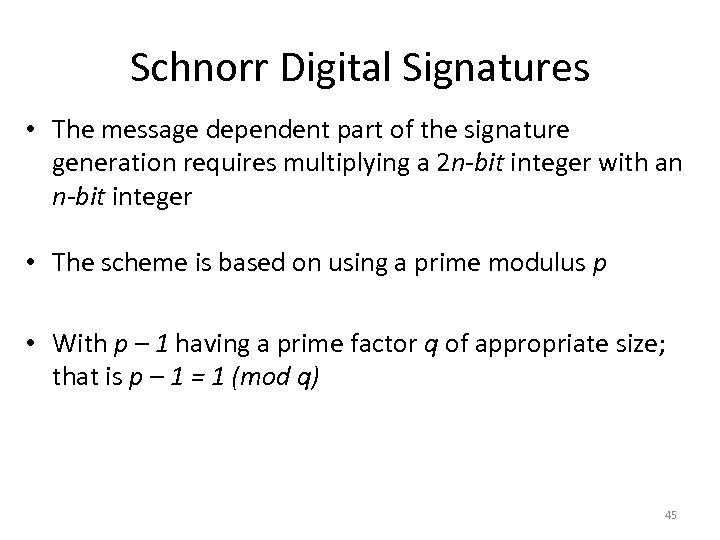Schnorr Digital Signatures • The message dependent part of the signature generation requires multiplying a 2 n-bit integer with an n-bit integer • The scheme is based on using a prime modulus p • With p – 1 having a prime factor q of appropriate size; that is p – 1 = 1 (mod q) 45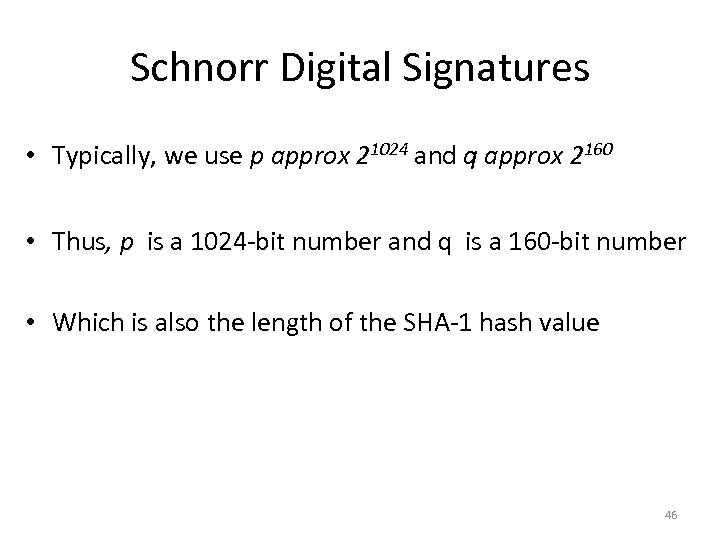Schnorr Digital Signatures • Typically, we use p approx 21024 and q approx 2160 • Thus, p is a 1024 -bit number and q is a 160 -bit number • Which is also the length of the SHA-1 hash value 46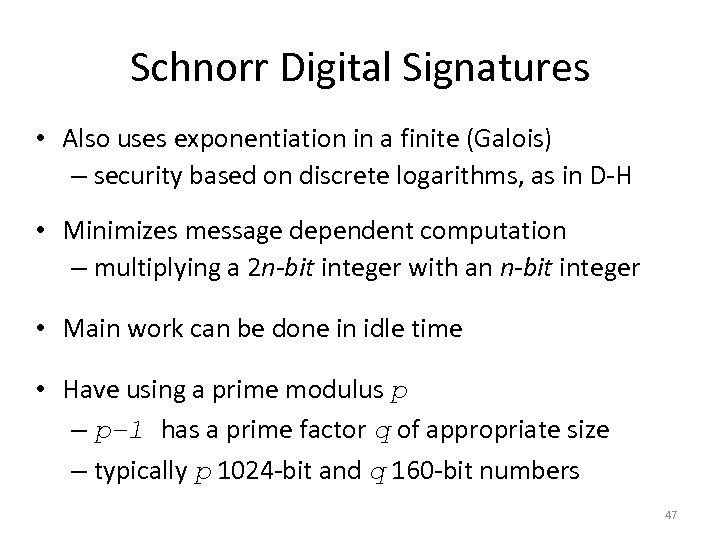Schnorr Digital Signatures • Also uses exponentiation in a finite (Galois) – security based on discrete logarithms, as in D-H • Minimizes message dependent computation – multiplying a 2 n-bit integer with an n-bit integer • Main work can be done in idle time • Have using a prime modulus p – p– 1 has a prime factor q of appropriate size – typically p 1024 -bit and q 160 -bit numbers 47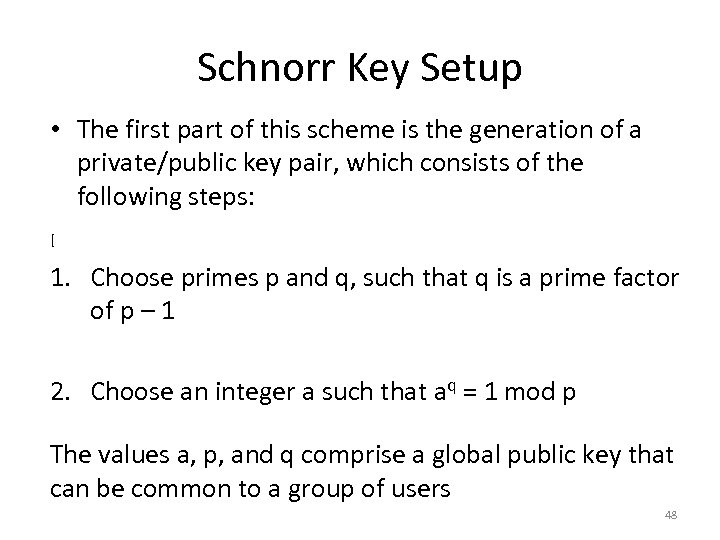Schnorr Key Setup • The first part of this scheme is the generation of a private/public key pair, which consists of the following steps: [ 1. Choose primes p and q, such that q is a prime factor of p – 1 2. Choose an integer a such that aq = 1 mod p The values a, p, and q comprise a global public key that can be common to a group of users 48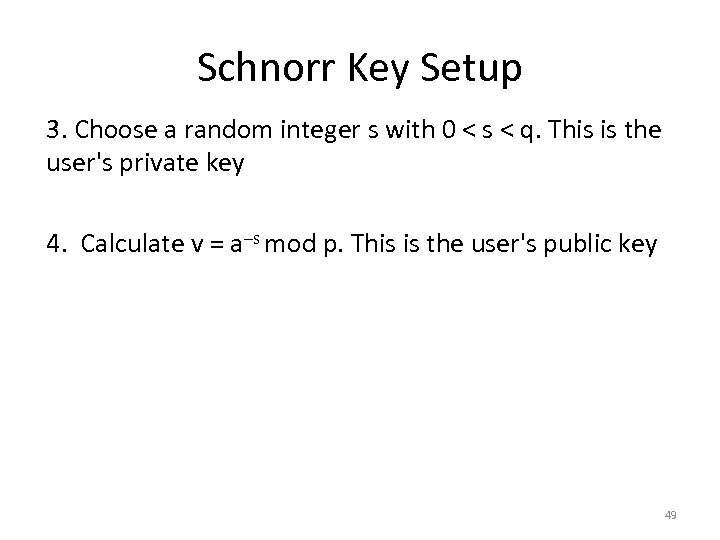Schnorr Key Setup 3. Choose a random integer s with 0 < s < q. This is the user's private key 4. Calculate v = a–s mod p. This is the user's public key 49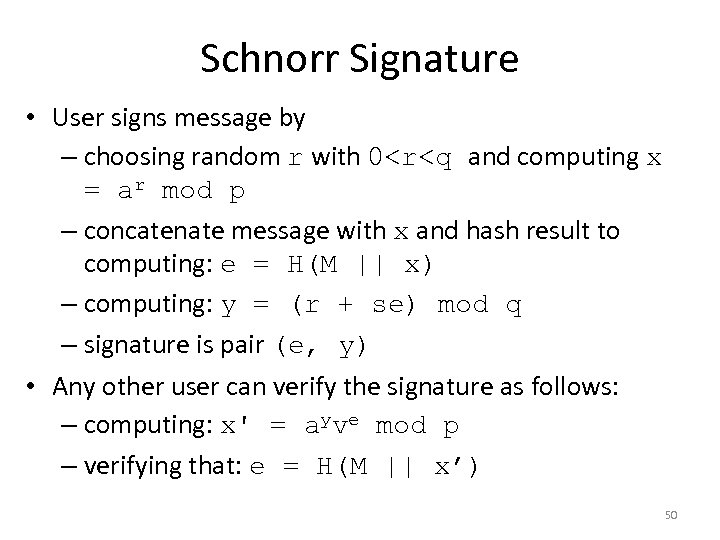Schnorr Signature • User signs message by – choosing random r with 0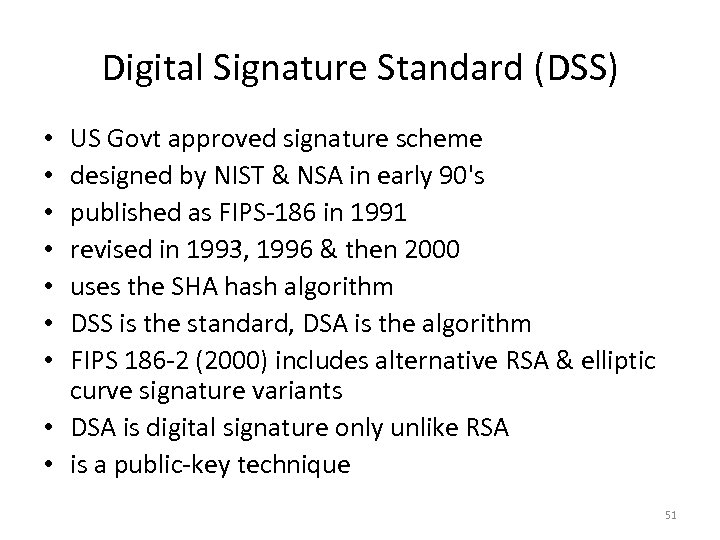Digital Signature Standard (DSS) US Govt approved signature scheme designed by NIST & NSA in early 90's published as FIPS-186 in 1991 revised in 1993, 1996 & then 2000 uses the SHA hash algorithm DSS is the standard, DSA is the algorithm FIPS 186 -2 (2000) includes alternative RSA & elliptic curve signature variants • DSA is digital signature only unlike RSA • is a public-key technique • • 51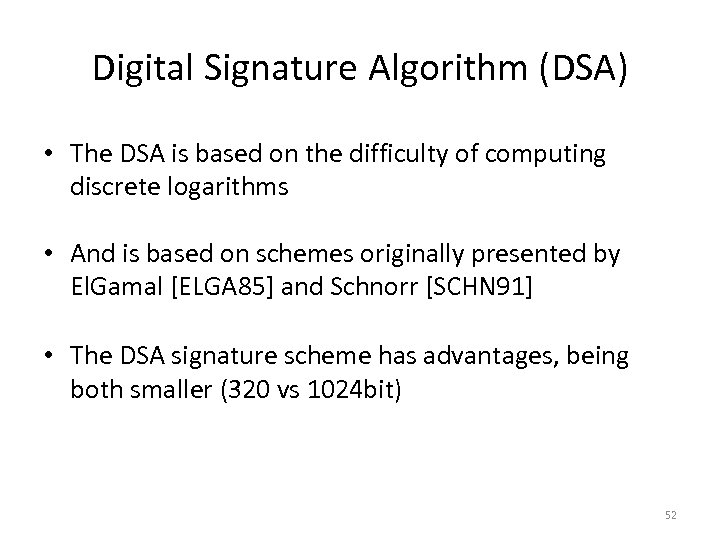Digital Signature Algorithm (DSA) • The DSA is based on the difficulty of computing discrete logarithms • And is based on schemes originally presented by El. Gamal [ELGA 85] and Schnorr [SCHN 91] • The DSA signature scheme has advantages, being both smaller (320 vs 1024 bit) 52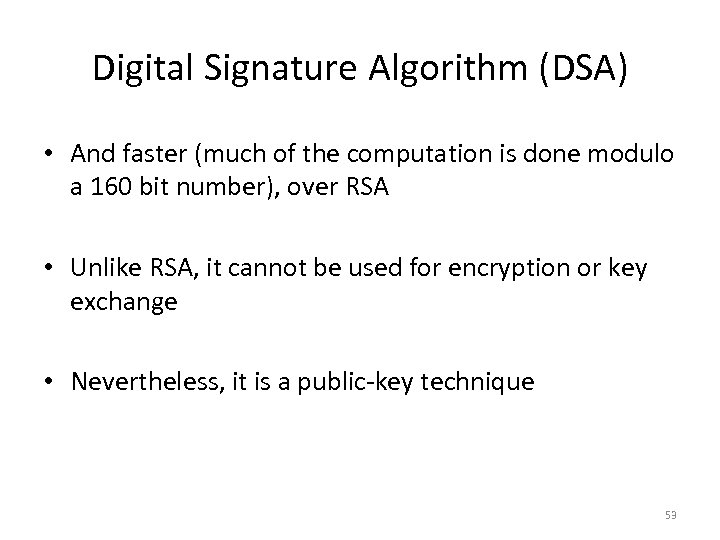Digital Signature Algorithm (DSA) • And faster (much of the computation is done modulo a 160 bit number), over RSA • Unlike RSA, it cannot be used for encryption or key exchange • Nevertheless, it is a public-key technique 53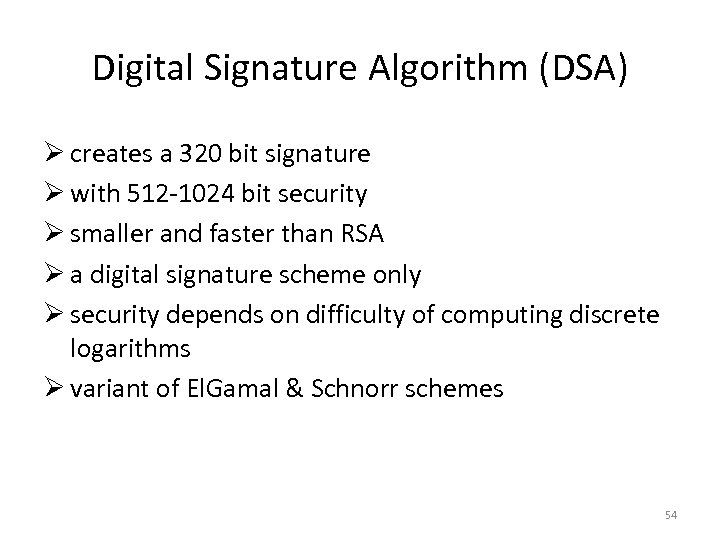Digital Signature Algorithm (DSA) Ø creates a 320 bit signature Ø with 512 -1024 bit security Ø smaller and faster than RSA Ø a digital signature scheme only Ø security depends on difficulty of computing discrete logarithms Ø variant of El. Gamal & Schnorr schemes 54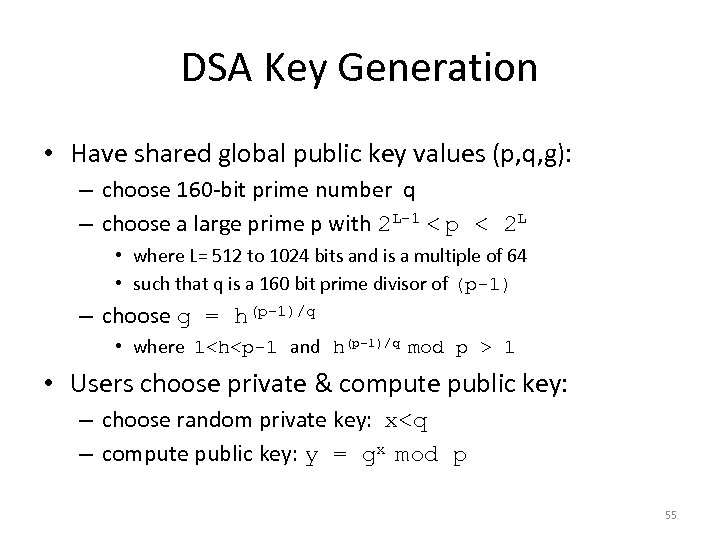DSA Key Generation • Have shared global public key values (p, q, g): – choose 160 -bit prime number q – choose a large prime p with 2 L-1 < p < 2 L • where L= 512 to 1024 bits and is a multiple of 64 • such that q is a 160 bit prime divisor of (p-1) – choose g = h(p-1)/q • where 1 1 • Users choose private & compute public key: – choose random private key: x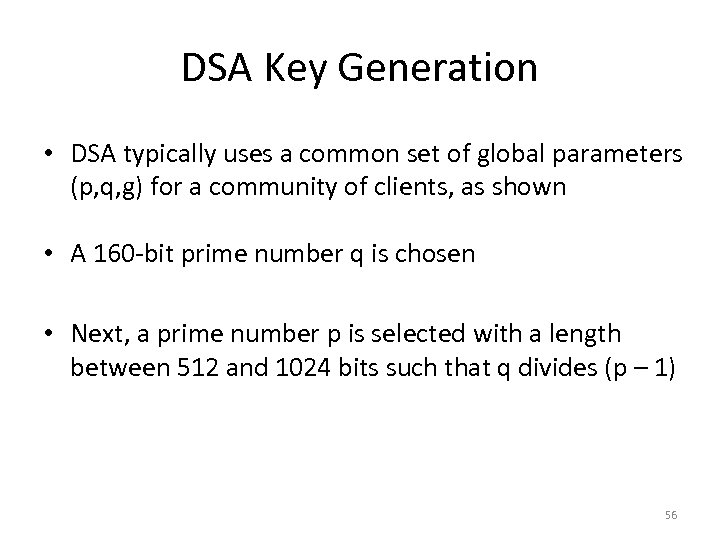DSA Key Generation • DSA typically uses a common set of global parameters (p, q, g) for a community of clients, as shown • A 160 -bit prime number q is chosen • Next, a prime number p is selected with a length between 512 and 1024 bits such that q divides (p – 1) 56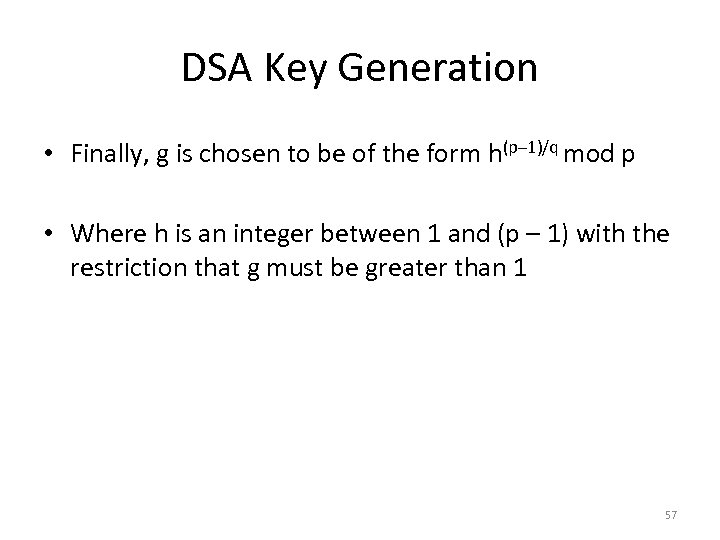DSA Key Generation • Finally, g is chosen to be of the form h(p– 1)/q mod p • Where h is an integer between 1 and (p – 1) with the restriction that g must be greater than 1 57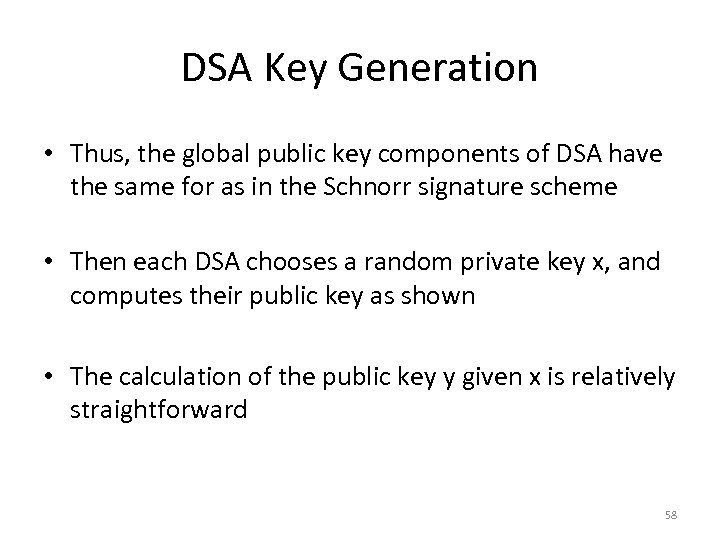DSA Key Generation • Thus, the global public key components of DSA have the same for as in the Schnorr signature scheme • Then each DSA chooses a random private key x, and computes their public key as shown • The calculation of the public key y given x is relatively straightforward 58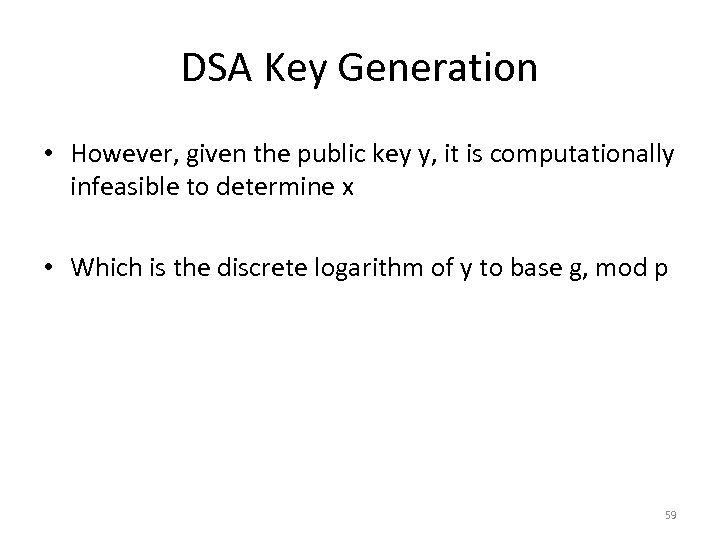DSA Key Generation • However, given the public key y, it is computationally infeasible to determine x • Which is the discrete logarithm of y to base g, mod p 59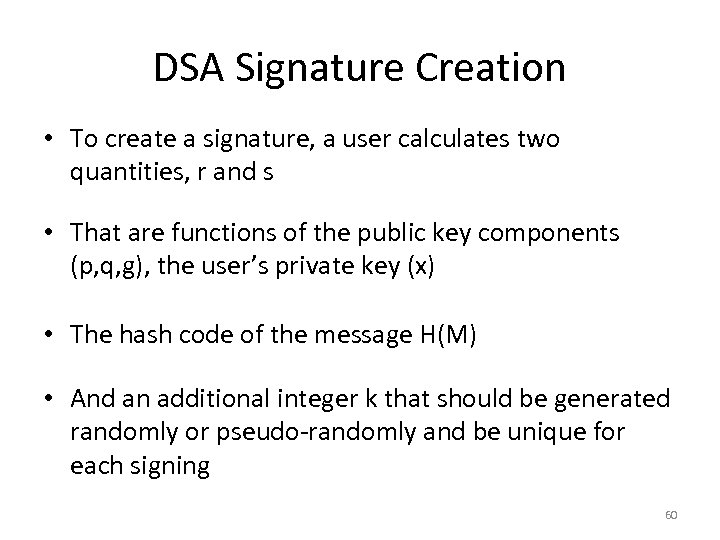DSA Signature Creation • To create a signature, a user calculates two quantities, r and s • That are functions of the public key components (p, q, g), the user’s private key (x) • The hash code of the message H(M) • And an additional integer k that should be generated randomly or pseudo-randomly and be unique for each signing 60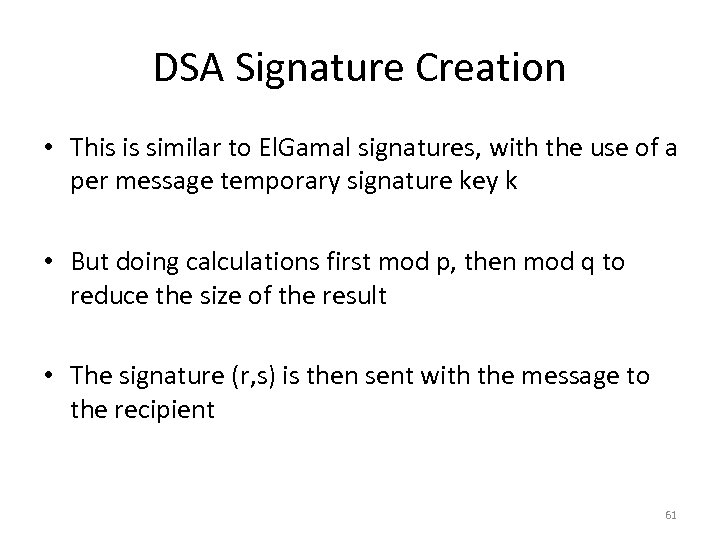DSA Signature Creation • This is similar to El. Gamal signatures, with the use of a per message temporary signature key k • But doing calculations first mod p, then mod q to reduce the size of the result • The signature (r, s) is then sent with the message to the recipient 61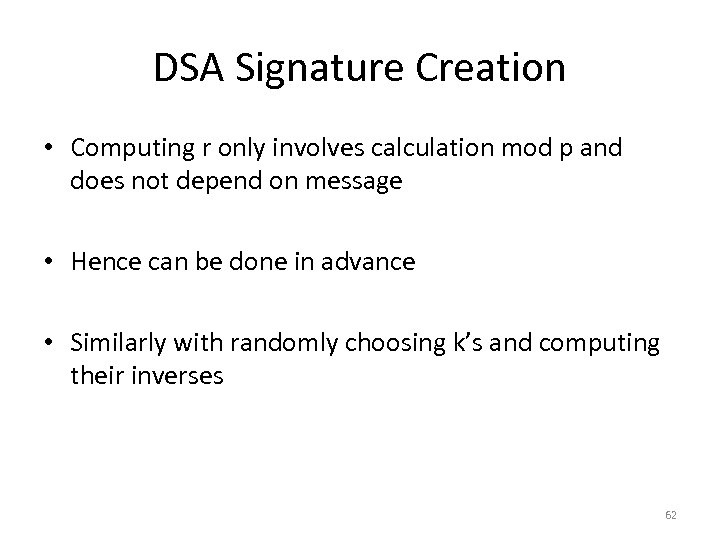DSA Signature Creation • Computing r only involves calculation mod p and does not depend on message • Hence can be done in advance • Similarly with randomly choosing k’s and computing their inverses 62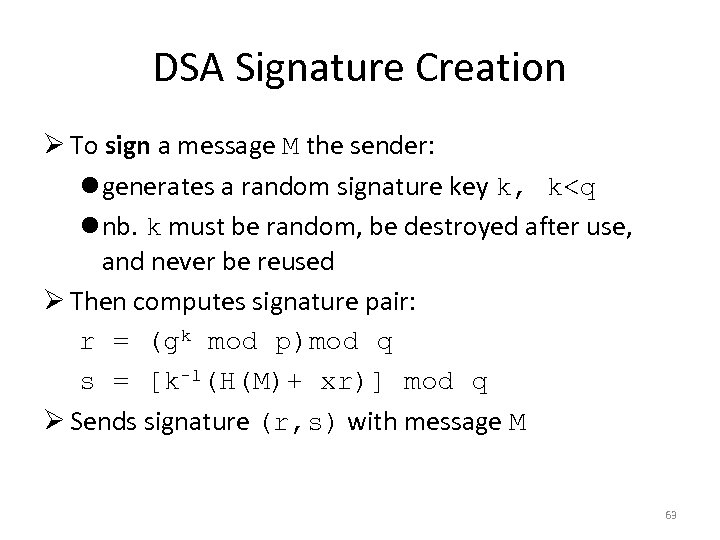DSA Signature Creation Ø To sign a message M the sender: l generates a random signature key k, k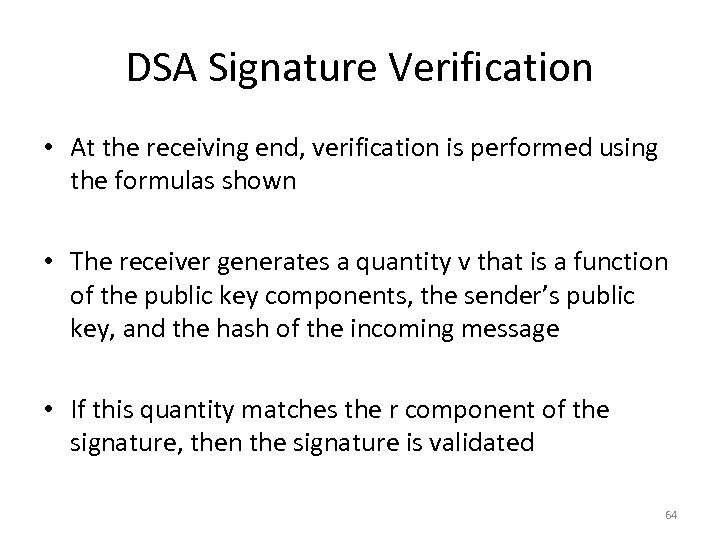DSA Signature Verification • At the receiving end, verification is performed using the formulas shown • The receiver generates a quantity v that is a function of the public key components, the sender’s public key, and the hash of the incoming message • If this quantity matches the r component of the signature, then the signature is validated 64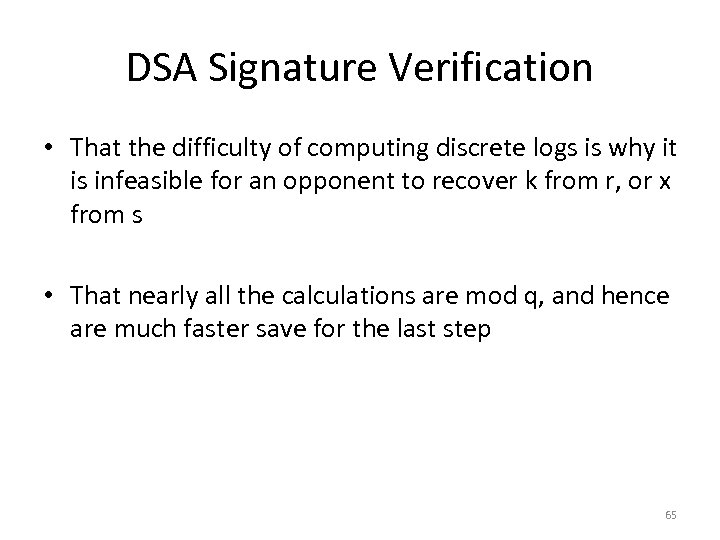DSA Signature Verification • That the difficulty of computing discrete logs is why it is infeasible for an opponent to recover k from r, or x from s • That nearly all the calculations are mod q, and hence are much faster save for the last step 65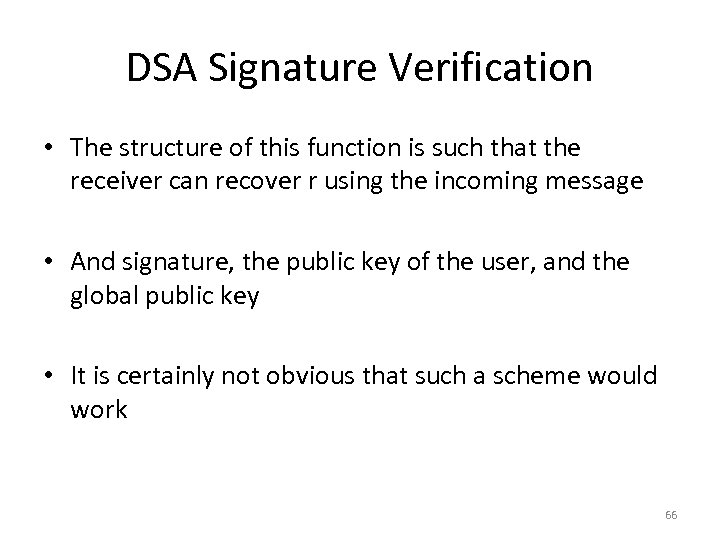DSA Signature Verification • The structure of this function is such that the receiver can recover r using the incoming message • And signature, the public key of the user, and the global public key • It is certainly not obvious that such a scheme would work 66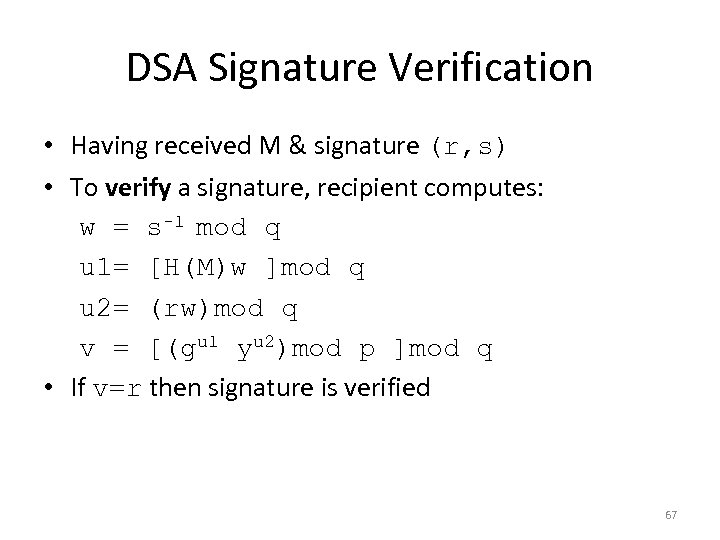DSA Signature Verification • Having received M & signature (r, s) • To verify a signature, recipient computes: w = s-1 mod q u 1= [H(M)w ]mod q u 2= (rw)mod q v = [(gu 1 yu 2)mod p ]mod q • If v=r then signature is verified 67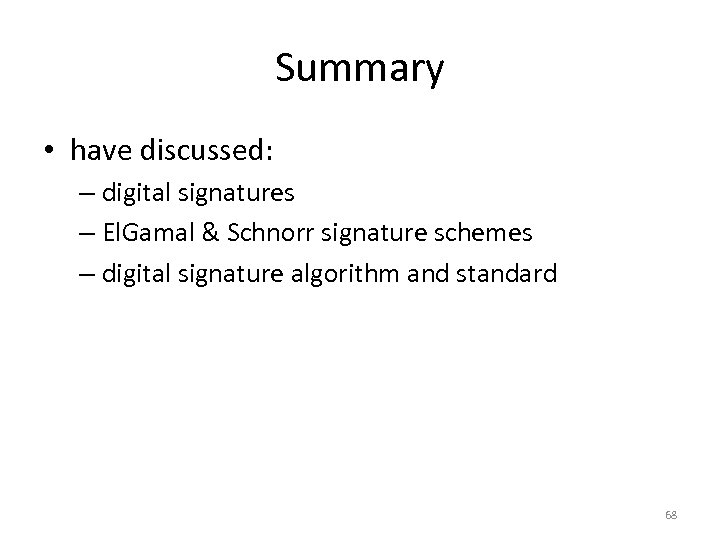Summary • have discussed: – digital signatures – El. Gamal & Schnorr signature schemes – digital signature algorithm and standard 68